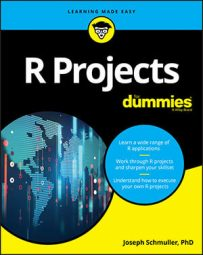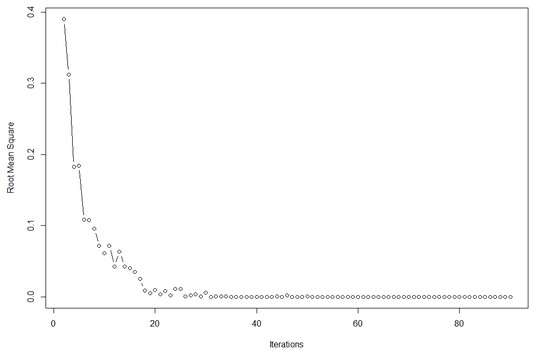##### R Projects For DummiesOne benefit of `Rattle` is that it allows you to easily experiment with whatever it helps you create with R. Here’s a little project for you to try. You’ll learn more about neural networks if you can see how the network error rate decreases with the number of iterations through the training set.

So the objective is to plot the error rate for the `banknote.uci` network as a function of the number of iterations through the training data. You should expect to see a decline as the number of iterations increases.

The measure of error for this little project is root mean square error (RMSE), which is the standard deviation of the residuals. Each residual is the difference between the network’s decision and the correct answer. You’ll create a vector that holds the RMSE for each number of iterations and then plot the vector against the number of iterations.

So the first line of code is

`rmse <- NULL`

Next, click the `rattle` Log tab and scroll down to find the R code that creates the neural network:

```crs\$nnet <- nnet(as.factor(Class) ~ .,
data=crs\$dataset[crs\$sample,c(crs\$input, crs\$target)],
size=3, skip=TRUE, MaxNWts=10000, trace=FALSE, maxit=100)```
The values in the `data` argument are based on Data tab selections. The `skip` argument allows for the possibility of creating skip layers (layers whose connections skip over the succeeding layer). The argument of most interest here is `maxit`, which specifies the maximum number of iterations.

Copy this code into RStudio.

Set `maxit` to `i`, and put this code into a `for`-loop in which `i` goes from 2 to 90.

The residuals are stored in `crs\$nnet\$residuals`. The RMSE is `sd(crs\$nnet\$residuals)`. Use that to update `rmse`:

`rmse <- append(rmse,sd(crs\$nnet\$residuals))`

So the general outline for the `for`-loop is

```for (i in 2:90){crs\$nnet <- create the neural net with maxit=i)
update the rmse vector }```
(This `for`-loop might take a few more seconds to run than you’re accustomed to.)

Finally, use the `plot()` function to plot RMSE on the y-axis and to plot iterations on the x-axis:

`plot(x=2:90, y=rmse, type="b", xlab="Iterations", ylab= "Root Mean Square")`

Your plot should look like this one.Root mean square error and iterations in neural networks for the `banknote.uci` data frame.

Here’s one more suggested project: Take another look at the code for creating `crs\$nnet`. Does anything suggest itself as something of interest that relates to RMSE? Something you could vary in a `for`-loop while holding `maxit` constant? And then plot RMSE against that thing? Go for it!